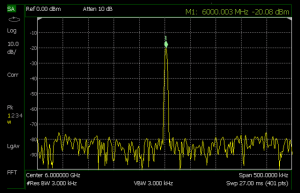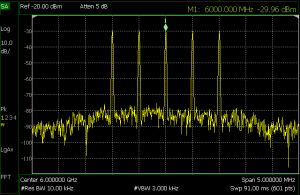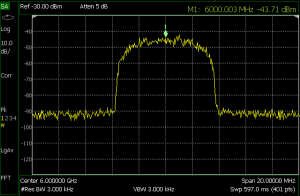# How to make good RF power measurements

The ability to make consistent measurements of RF or microwave power levels is important in the development of radio systems. Making measurements requires an informed choice of equipment and approach, to ensure that the limitations of each does not undermine the validity of the measurements.

Let us compare two different approaches; one using a power sensor and the other a spectrum analyser, to make average power measurements of continuous wave (CW), multi-tone, 32 QAM (quadrature amplitude modulation), and pulsed signals.

The test signals came from a vector signal generator producing a 6GHz signal with an output power of -20dBm.

The power sensor measurements used a USB power sensor (Keysight U2000A) connected to an N9938A FieldFox spectrum analyser with Option 302.Figure 1

The spectrum-analyser based approach used the same Fieldfox system, configured with a built-in channel power meter (CPM), connected to the signal generator over coaxial cable.

Measuring a CW signal

CW signals do not carry any information and so the average power and peak power are the same and the signal has no bandwidth. This means that measurement equipment can use a narrow filter to improve its sensitivity (noise floor).

Figure 1 shows the swept frequency response of a typical spectrum analyser, around a single tone across a measurement span of 500kHz. The signal’s peak power (which is also its average power) is given as -20.08dBm.

The average power measured by the USB power sensor was -20.00dBm. The average power measured using the spectrum analyser was -20.09dBm.

The three measurements are within 0.1dB of each other, with the power sensor having the highest accuracy, or lowest measurement uncertainty.

The analyser’s built-in power measurement system has to have a span set for it. This is equivalent to the channel bandwidth of the signal under test. In the analyser measurement, the span was set to 100kHz. Since a CW signal has no bandwidth, the span can be set to any value, although a narrow span increases measurement time while a wider span increases noise and uncertainty.

Measuring a multi-tone signal

In this example, the power from the signal generator is distributed among five tones, spaced at 500kHz intervals.Figure 2

If the total power for the tones is -20dBm (10μW) then each has a power of ‑26.98dBm (2μW).

An average power sensor will measure the average power of all five tones, in this case, -20.13dBm. The analyser with power meter option gives a measurement of ‑20.2dBm, so long as the power meter’s measurement span has been set to include all five tones (a span of at least 2.5MHz, in this case). (See Figure 2)

If this measurement was repeated with a span of just 200kHz, only the central tone at 6GHz would be included, giving a power measurement of -27.1dBm — close to the theoretical value of -26.98dBm for a single tone.

Making measurements of single tones or specific measurement spans is only possible with a spectrum analyser, since power sensors are not frequency selective.

The peak power of this signal is not the same as its average power, because of the phase relationship between the tones. Peak power measurements usually need a sensor with peak power capability and a filter wide enough to process all the tones at once.

Measuring a digitally modulated signal

The digitally modulated signal shown here uses 32 QAM with a symbol rate of 5Msymbol/s. The symbols are filtered, so the signal bandwidth is approximately 8MHz. The power sensor reports an average power of -20.00dBm and the analyser power meter reports -20.0 7dBm.

A power sensor will report the correct average power of a signal with unknown bandwidth so long as the signal’s power level and frequency range are within the sensor’s capabilities.Figure 3

On the other hand, the correct measurement span must be set when using the analyser to make accurate measurements. To do this, you can use a standard spectrum analyser to discover the bandwidth of the unknown signal, and then switch back to the channel power meter once it is configured with the span setting to match.

Measuring pulsed waveforms

In pulsed waveforms, the ratio of the pulse width (how long the pulse is on) to the time between the pulses (its period) is known as the duty factor (DF). The peak power of such waveforms is the average power divided by the DF, given a repeating pulse-train.

Figure 4 shows an example of the measured spectrum and average power of a pulsed waveform with a 20μs pulse width and a 20% DF.

The spectrum analyser display in Figure 4 shows a typical “sin(x)/x” response as a function of frequency. The sidebands in the frequency domain continue outside the displayed 1MHz frequency span. The average power measurement using the power sensor reports a power of -27.01dBm. The measurement using the analyser power meter reports an average power of –26.8dBm.

For the analyser measurement, the span was set to 3MHz to capture most of the waveform’s sideband energy. Increasing the span to more than 3MHz did not change the measured power.

The peak power for this waveform with a 20% DF can be calculated by increasing the average level by 6.99dB (10log(1/DF)). Using a measured average power of -27dBm, the peak power is calculated to be -20dBm — which is what the signal generator was set to produce.

Detection and filtering in peak power measurements

The block diagram for a power sensor and meter designed to measure peak power is similar to that for an average power sensor. The peak power sensor/meter will have a wider bandwidth and higher sample rates in its ADC, to capture the rapid transitions found in pulsed and complex modulated waveforms.

In practice, a typical peak power sensor has two measurement paths, optimised for measuring either the average power or the peak power.

In the peak power measurement path, the sensor has a two-layer detection scheme.Figure 4

The first detection occurs at the front end where the diode rectifies the incoming waveform. As the diode detector is usually a wideband device, the rapidly changing envelope of the pulsed waveform is preserved in the rectified waveform.

After detection, the signal is amplified and filtered. The filter’s bandwidth is likely to be selectable, at up to 30MHz, and has to be greater than the signal under measurement.

The second detection layer occurs after filtering, where the ADC samples the signal at up to 1Gsample/s. This sampling rate is necessary to capture the shape of the waveform, from which the peak power, pulse width, period, rise time, and fall time characteristics can be measured or derived.

Peak power sensors can also interface with power meters, spectrum analysers, and laptops for field measurements. The next section shows how a peak power sensor connected to a portable spectrum analyser can be used to measure the time domain profile of a pulsed waveform.

Making measurements with a peak power sensor

The input waveform is a pulse-modulated 40GHz signal with a 1μs pulse width and 10% DF. It was captured using a handheld spectrum analyser connected to a USB peak and average power sensor. In this set-up, only the power sensor needs to be rated to 40GHz, as the spectrum analyser is only used as a display and control interface.

Using this type of peak power sensor, an analyser or peak power meter can display peak power, average power, and waveform characteristics such as rise and fall times.

Modern equipment, be it a standalone power sensor or power meter, or a spectrum analyser with CPM, can make sophisticated measurements on complex signals. However, there are nuances to the way each works that need to be understood if the user is going to be confident in the measurements made in each context.

Giovanni D’Amore, Marketing Brand Manager, Keysight Technologies